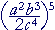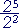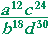S k i l l
i n
A L G E B R A

21

# NEGATIVE EXPONENTS

Power of a fraction

Section 2

Power of a fraction"To raise a fraction to a power, raise the numerator
and denominator to that power."

 Example 1.For,  according to the meaning of the exponent, and the rule for multiplying fractions:Example 2.   Apply the rules of exponents:Solution.   We must take the 4th power of everything.  But to take a power of a power -- multiply the exponents:Problem 1.   Apply the rules of exponents.

To see the answer, pass your mouse over the colored area.
To cover the answer again, click "Refresh" ("Reload").
Do the problem yourself first!

 a)= x2y2 b)= 8x327 c)=d)=e)= x2 − 2x + 1x2 + 2x + 1

Perfect Square Trinomial

Subtracting exponents

In the previous Lesson we saw the following rule for reducing a fraction:

 axay = xy

"Both the numerator and denominator may be divided
by a common factor."

Consider these examples:

 2· 2· 2· 2· 2     2· 2 = 2· 2· 2
 ___2· 2___2· 2· 2· 2· 2 = __1__2· 2· 2

If we write those with exponents, then22 = 23
 2225 = 1 23

In each case, we subtract the exponents.  But when the exponent in the denominator is larger, we write 1-over the difference.

 Example 3.x3 = x5x8 = 1 x5

Here is the rule:Problem 2.   Simplify the following.  (Do not write a negative exponent.)

 a)= x3 b) x2x5 = 1 x3 c) xx5 = 1x4
 d) x2x = x e)= −x4 f)= 1x2

Problem 3.   Simplify each of the following.  Then calculate each number.

 a)= 23 = 8 b) 2225 = 1 23 = 18 c) 225 = 124 = 1 16
 d) 222 = 2 e)= −24 = −16. See Lesson 13.
 f)= 122 = 14
 Example 4.   Simplify by reducing to lowest terms:Solution.   Consider each element in turn:Problem 4.   Simplify by reducing to lowest terms.  (Do not write negative exponents.

 a)= y35x3 b) b)= − 8a35b3
 c)= − 3z_5x4y3 d)= c316
 e) (x + 1)3 (x − 1)(x − 1)3 (x + 1) = (x + 1)2(x − 1)2

Negative exponents

We are now going to extend the meaning of an exponent to more than just a positive integer.  We will do that in such a way that the usual rules of exponents will hold.  That is, we will want the following rules to hold for any exponents:  positive, negative, 0 -- even fractions.

 aman = am + n Same Base (ab)n = anbn Power of a Product (am)n = amn Power of a Power

We begin by defining a number with a negative exponent.

 a−n = 1 an

It is the reciprocal of that number with a positive exponent.

an is the reciprocal of an.

 Example 5. 2−3 = 123 = 18

The base, 2, does not change.  The negative exponent becomes positive -- in the denominator.

Example 6.   Compare the following. That is, evaluate each one:

3−2    −3−2   (−3)−2    (−3)−3

 Answers. 3−2 = 132 = 19 .

Next,

−3−2 is the negative of 3−2. (See Lesson 13.)  The base is still 3.

 −3−2 = − 19 .

As for (−3)−2, the parentheses indicate that the base is −3:

 (−3)−2 = 1   (−3)2 = 19 .

Finally,

 (−3)−3 = 1   (−3)3 = − 1 27 .

A negative exponent, then, does not produce a negative number.  Only a negative base can do that. And then the exponent must be oddExample 7.   Simplify a2a5 .

Solution.   Since we have invented negative exponents, we can now subtract any exponents as follows:

 a2a5 = a2 − 5 = a−3

We now have the following rule for any exponents m, n:In fact, it was because we wanted that rule to hold that we

 defined a− n as 1 an .

We want

 a2a5 = a−3

But

 a2a5 = 1 a3
 Therefore, we define  a−3 as 1 a3 .
 Example 8. a−1 = 1a

a−1 is now a symbol for the reciprocal, or multiplicative inverse, of any number a.  It appears in the following rule (Lesson 5):

a· a−1 = 1

Problem 5.   Evaluate the following.

 a)  ( 23 )−1 = 32 . 32 is the reciprocal of 23 .
 b)  ( 23 )−4 = 8116 . 8116 is the reciprocal of the 4th power of 23 .
 Problem 6.   Show:  am· b−n  = ambn .
 am· b−n  =  am· 1bn = ambn . The definition of division.

Example 9.   Use the rules of exponents to evaluate  (2−3· 104)−2.

 Solution. (2−3· 104)−2 = 26· 10−8 Power of a power =Problem 6 = _64_      100,000,000

Problem 7.   Evaluate the following.

 a) 2−4 = 1 24 = 116 b) 5−2 = 1 52 = 125 c) 10−1 = 1 101 = 110
 d) (−2)−3 = 1   (−2)3 = 1  −8 = − 18
 e) (−2)−4 = 1   (−2)4 = 1 16 f) −2−4 = − 1 24 = − 1 16

g)  (½)−1 =  2.   2 is the reciprocal of ½.

Problem 8.   Use the rules of exponents to evaluate the following.

 a) 102· 10−4 = 102 − 4 = 10−2 = 1/100.
 b) (2−3)2 = 2−6 = 1 26 = 1 64
 c) (3−2· 24)−2 = 34· 2−8 = 3428 = 81 256
 d) 2−2· 2 = 2−2+1 = 2−1 = 12

Problem 9.   Rewrite without a denominator.

 a) x2x5 = x2−5 = x−3 b) yy6 = y1−6 = y−5
 c)= x−3y−4 d)= a−1b−6c−7
 e) 1x = x−1 f) 1x3 = x−3
 g) (x + 1)    x = (x + 1)x−1 h) (x + 2)2(x + 2)6 = (x + 2)−4

Example 10.     Rewrite without a denominator, and evaluate:Answer.   The rule for subtracting exponents ---- holds even when an exponent is negative.Therefore,= 10−3 + 5 − 2 + 4 = 104 = 10,000.

Exponent 2 goes into the numerator as −2;  exponent −4 goes there as +4.

Problem 10.    Rewrite without a denominator and evaluate.

 a) 222−3 = 22 + 3  = 25 = 32 b) 10210−2 = 102 + 2  = 104 = 10,000
 c)= 102 − 5 − 4 + 6  = 10−1 = 1 10
 d)= 25 − 6 + 9 − 7  = 21 = 2

The reciprocal of an.

Reciprocals come in pairs.  The reciprocal of  an  is  an :

 1 an = a−n .

And the reciprocal of  an  is  an :

 1  a−n = an .

That implies:

Factors may be shifted between the denominator and the numerator
by changing the sign of the exponent.

 Example 11.   Rewrite without a denominator:Answer.The exponent 3 goes into the numerator as −3;  the exponent −4 goes there as +4.

Problem 11.    Rewrite with positive exponents only.

 a) x y−2 = xy2 b)=c)=d)=e)=Problem 12.    Apply the rules of exponents, then rewrite with positve exponents.

 a)==b)==Section 2: Exponent 0Next Lesson:  Multiplying and dividing algebraic fractions

Please make a donation to keep TheMathPage online.
Even \$1 will help.